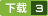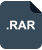混合编程——C++调用MATLAB程序 评分:

2018-11-21 上传大小：18.49MBC++ MATLAB 混合编程——VS项目调用MATLAB函数c++调用matlab函数 - dll方式C++调用matlab接口C/C++ VS中调用matlab函数的方法C/C++ 中调用matlab 的两种方式C++调用MATLAB函数C++调用matlab代码C++调用MATLAB函数、打包dllwindows下C++如何调用matlab程序matlab函数编译成库供C++调用（非常详细）c++调用matlab程序C++调用MATLAB程序，以及常用问题解决C/C++调用matlab程序MATLAB调用C++程序c++调用matlab代码 立即下载C/C++和matlab混合编程Matlab和C混合编程matlab 和 C++混合混合编译及调试qq_1196581964热点文章

• C++ MATLAB 混合编程——VS项目调用MATLAB函数

2018-06-28 wokaowokaowokao12345
• c++调用matlab函数 - dll方式

2018-06-09 u013735511
• c++调用matlab引擎solve()函数时，无法从matlab中读取正确结果

2016-12-04 qq_32353953
• C++调用matlab接口

2019-04-22 Aidam_Bo
• C/C++ VS中调用matlab函数的方法

2017-07-01 guyuealian
• C/C++ 中调用matlab 的两种方式

2016-04-20 u013575812
• C++调用MATLAB函数

2019-03-15 weixin_41923961
• C++调用matlab代码

2019-01-09 xswgongchenshi
• C++调用MATLAB函数、打包dll

2019-06-04 qq_34037046
• 使用c++调用matlab的问题

2010-06-26 wangxiaojun911

公告

下载码下载spring mvc+mybatis+mysql+maven+bootstrap 整合实现增删查改简单实例.zip

 资源所需积分/C币 当前拥有积分 当前拥有C币 5 0 0VIP下载

积分不足！

 资源所需积分/C币 当前拥有积分

 4000万 程序员的必选 600万 绿色安全资源 现在开通 立省522元资源所需积分/C币 当前拥有积分 当前拥有C币 5 4 45资源所需积分/C币 当前拥有积分 当前拥有C币 5 0 0资源所需积分/C币 当前拥有积分 当前拥有C币 5 4 45

• 举报人：
• 被举报人：
• *类型：
• *投诉人姓名：
• *投诉人联系方式：
• *版权证明：
• *详细原因：